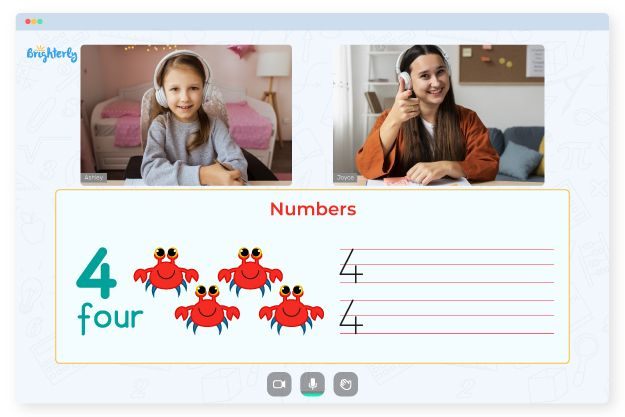# 2nd Grade Math Facts Worksheets

A child’s mathematical development in the second grade aims to provide a kid with a solid foundation in math facts. It is a significant developmental stage. Children must memorize addition, subtraction, multiplication, and division facts, which are fundamental calculations.

Students should have a solid understanding of addition and subtraction facts when they enter second grade. With the assistance of a Multiplication Chart, Multiplication Table, or other memory aid, many students in the second grade will be prepared to begin working on early multiplication math fact worksheets 2nd grade.

The addition and subtraction fact family worksheets 2nd grade are among the most common types of second-grade math facts worksheets. The purpose of these worksheets is to teach students how to quickly and accurately add and subtract numbers.

Multiplication and division doubles facts worksheets 2nd grade are other types of second-grade math facts worksheets. These worksheets help students learn how to multiply and divide numbers quickly and accurately.

Math for Kids

Is Your Child Struggling With Math?
1:1 Online Math Tutoring## Benefits

The second grade math facts worksheets are designed to help students learn math fundamentals enjoyably and excitingly. Teachers can use these worksheets for various purposes, including review for exams, in-class activities, and homework assignments.

Fact families worksheets 2nd grade are a valuable resource for both teachers and students. Students can learn and practice their math skills in a fun and engaging way and get immediate feedback that helps them see where they need to improve.### 2nd Grade Math Facts Worksheets PDF### 2nd Grade Math Facts Worksheets PDF### 2nd Grade Math Facts Worksheets PDF### 2nd Grade Math Facts Worksheets PDF

Need help with Numbers?• Does your child need help with math numbers?
• Consider starting lessons with an online tutor.

Is your child struggling with understanding the concept of numbers? Try learning with an online tutor.